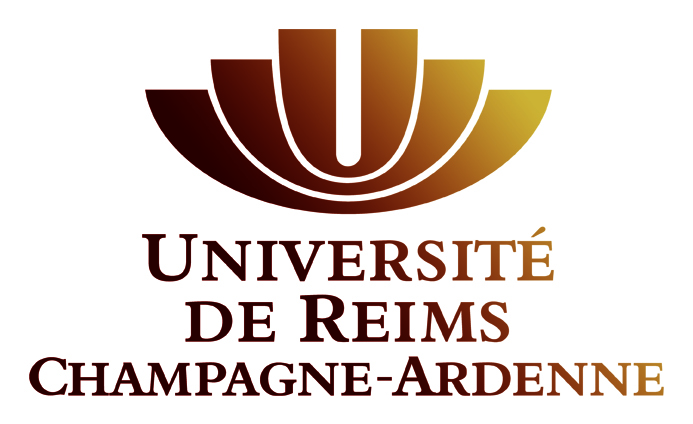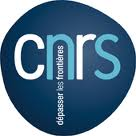Journées SL(2,R)
Reims, 20-21 novembre 2014

# Journées « Théorie des représentations des groupes de Lie » Strasbourg-Lorraine-Luxembourg-Reims

## Organisateurs

• Michael Pevzner
• Rupert Yu
L'inscription est gratuite mais obligatoire pour des raisons d'organisation. Si vous souhaitiez participer à ces journées, veuillez contacter au plus tard le 7 novembre l'un des organisateurs par e-mail à l'adresse suivante :

prenom.nom(arobase)univ-reims.fr

## Conférenciers

• W. Bertram (Nancy)
• V. Fischer (Londres)
• G. Liu (Metz)
• V. Molchanov (Tambov)
• M. Olbrich (Luxembourg)
• L. Poulain d'Andecy (Reims)
• H. Rubenthaler (Strasbourg)
• V. Tsanov (Göttingen)

## Information pratique

• Venir au Campus Moulin de la Housse - Plan (pdf)
• Liste des participants

## Programme

Les cours auront lieu dans la Salle de Séminaire du Département MMI - bâtiment 3 du Campus Moulin de la Housse.

 Jeudi 20 novembre 13h30 Accueil 14h-14h50 H. Rubenthaler (Strasbourg) Graded Lie algebras associated to a representation of a quadratic algebra Let $$\mathfrak{g}_{0}$$ be a quadratic Lie algebra (i.e. a Lie algebra with a non-degenerate symmetric invariant bilinear form. Let $$(\mathfrak{g}_{0},\rho, V)$$ be a finite dimensional representation of $$\mathfrak{g}_{0}$$. We associate to such a representation a graded Lie algebra $$\mathfrak{g}=\bigoplus_{n\in \mathbb{Z}} \mathfrak{g}_{n}$$, where $$\mathfrak{g}_{0}$$ is the given algebra, where $$\mathfrak{g}_{1}=V$$, $$\mathfrak{g}_{-1}=V^{*}$$, and where the bracket between $$\mathfrak{g}_{0}$$ and $$\mathfrak{g}_{1}$$ (resp. $$\mathfrak{g}_{0}$$ and $$\mathfrak{g}_{-1}$$) is the representation $$\rho$$ (resp. the contragredient representation $$\rho^{*}$$). In fact several such graded algebras exist. We will investigate these graded Lie algebras, more specifically in the case where $$\mathfrak{g}_{0}$$ is reductive and we will give several applications. 15h-15h50 L. Poulain d'Andecy (Reims) Representations of affine Hecke algebras of type $$D_{n}$$ and Young tableaux I will present a simple/naive generalisation of the usual notion of standard Young tableaux, and explain how to use them to express the classification of some modules over an affine Hecke algebra of type D. I will start with recalling known results about affine Hecke algebras of type A and their cyclotomic quotients, in order to give some motivations for this work. Pause café 16h20-17h10 V. Tsanov (Göttingen) Geometric branching laws We consider the branching law for a pair of semisimple complex Lie groups $$G < G'$$, that is, the decompositions of irreducible $$G'$$-modules into irreducible $$G$$-submodules. Specifically, we focus on a correspondence between certain $$G$$-submodules and certain $$G$$-orbits in the flag varieties of $$G'$$. The main tools we use are the Borel-Weil-Bott theorem, its Lie-algebraic counterpart due to Kostant and the Belkale-Kumar product in cohomology of flag varieties. 17h20-18h10 G. Liu (Metz) Homologie des algèbres de Lie à valeurs dans un espace de Schwartz et théorème de comparaison. Dans cet exposé, je parlerai de la propriété de séparation de Hausdorff pour l'homologie des algèbres de Lie à valeurs dans un espace de Schwartz, ainsi que de son lien avec un « théorème » (plutôt une conjecture) de comparaison. Il s'agit d'un travail en commun avec A. Aizenbud, D. Gourevitch et B. Krötz. Dîner Vendredi 21 novembre 9h-9h50 W. Bertram (Nancy) Touching an associoid I intend to present a combination of research topics which issues related to teaching mathematics (at high school and university level): on the research side, the concept of "associoid" is a general version of associative structures, comprising groups, groupoids, torsors, principal bundles..., and I will try to explain that this framework is appropriate for better understanding the "associative geometries" introduced by Kinyon and myself (see http://arxiv.org/abs/1406.1692 ). On the pedagogical side, dynamical geometry software "geogebra" is a very interesting tool for teaching mathematics, as I will try to illustrate by showing dynamical images of (non-commutative!) torsors - see http://arxiv.org/abs/1305.6851 for a static version. 10h-10h50 M. Olbrich (Luxembourg) Compact quotients of Cahen-Wallach spaces A venir. Pause café 11h10-12h V. Fischer (Londres) Pseudo-differential operators and quantization on Lie groups A venir. 12h10-13h V. Molchanov (Tambov) Poisson and Fourier transforms for tensor products A venir.